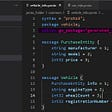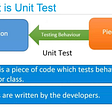# Zk-SNARKs: Under the Hood

• A is a linear combination of a set of polynomials {A_1…A_m}
• B is the linear combination of {B_1…B_m} with the same coefficients
• C is a linear combination of {C_1…C_m} with the same coefficients
• G * A_1(t), G * A_1(t) * k_a
• G * A_2(t), G * A_2(t) * k_a
• G * B_1(t), G * B_1(t) * k_b
• G * B_2(t), G * B_2(t) * k_b
• G * C_1(t), G * C_1(t) * k_c
• G * C_2(t), G * C_2(t) * k_c
• π_a = G * A(t), π’_a = G * A(t) * k_a
• π_b = G * B(t), π’_b = G * B(t) * k_b
• π_c = G * C(t), π’_c = G * C(t) * k_c
1. decrypt(old_balance, k) ≥ decrypt(tx_value, k)
2. decrypt(old_balance, k) - decrypt(tx_value, k) = decrypt(new_balance, k)
• Dividing (A * B - C) / Z to get H (algorithms based on the Fast Fourier transform can do this in sub-quadratic time, but it’s still quite computationally intensive)
• Making the elliptic curve multiplications and additions to create the A(t), B(t), C(t) and H(t) values and their corresponding pairs

--

--

--

## More from Vitalik Buterin

Love podcasts or audiobooks? Learn on the go with our new app.

## JBoss — Sensitive Information Disclosure | PoC | Lucideus Research## Part 1: Grpc Proto Code Generation Using Protoc for Message## Top Productivity Tools of July on BetaPage That You Must Use## Modal: Design for Enterprise — Part II## You have to read this comparison before you will buy another online programming course!## Unit Testing, How and Why ?## A month in review. . . and looking ahead## Implementing the Health Check API Pattern with Rust## Curve Finance and veCRV## Warp Finance announces completion of Warp V2 audit by Stela Labs## Introducing Galleon DAO — DeFi Structured Products Methodologists & 17th-Century Roleplay